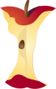Mental Addition and Subtraction of 10s or 100sPrintable Worksheets And Lessons

• Step-by-step Lesson- I broke out the numbers line to help us. We move between the addition and subtraction of 10s.

• Guided Lesson - The focus is on on the movement between tens place again here.

• Guided Lesson Explanation - Sorry, I might have gone a bit overboard with explaining here. I just wanted to make sure a students wouldn't need any outside help.

• Practice Worksheet - Ten problems that all use a numbers line to prompt the students.

• Matching Worksheet - Match the missing numbers line spot to the number that is missing.(Click Here to Upgrade)

Homework Sheets

Fill in the partially missing parts of the numbers line.

Practice Worksheets

You will start with blank number lines; except for the start and finish.

Math Skill Quizzes

Remember to make a mental note of the type of operations that are going on here.

 Quiz 1 Quiz 2 Quiz 3